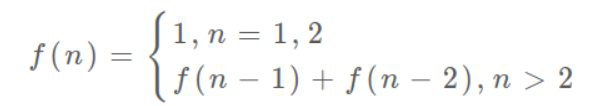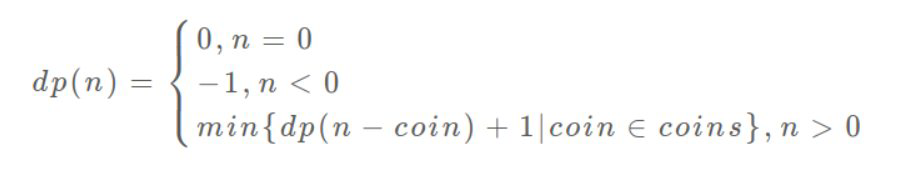# 动态规划（DP）

• 首先，动态规划的穷举有点特别，这类问题存在「重叠子问题」，穷举的话效率会极其低下，所以需要缓存来优化穷举过程，避免不必要的计算。

• 其次，动态规划问题一般有「最优子结构」，用子问题的最优解获得整个问题的最优解。

• 另外虽然动态规划的核心思想就是穷举求最值，但是问题可以千变万化，只有列出正确的「状态转移方程」才能正确地穷举。

### 斐波那契数列

``````    public function dp(\$number)
{
if(\$number==1||\$number==2){
return 1;
}
return \$this->dp(\$number - 1) + \$this->dp(\$number- 2);
}``````

``````class SequenceDP
{
public \$array;

public function __construct(\$number)
{
for (\$i = 0; \$i < \$number + 1; \$i++) {
\$this->array[\$i] = -1;
}
}

public function dp(\$number)
{
if(\$number==1||\$number==2){
return 1;
}
if ( \$this->array[\$number] != -1)
return \$this->array[\$number];
return \$this->dp(\$number - 1) + \$this->dp(\$number- 2);
}
}

\$xx = new SequenceDP(10);
\$int = \$xx->dp(10);
var_dump(\$int);````````````function fib(\$n) {
if (\$n == 2 || \$n == 1)
return 1;
\$prev = 1, \$curr = 1;
for (\$i = 3;\$i <=\$n; \$i++) {
\$sum = \$prev + \$curr;
\$prev = \$curr;
\$curr = \$sum;
}
return \$curr;
}``````

### 凑零钱的问题

``````# 要凑出金额 n，至少要 dp(n) 个硬币
function dp(n){
# 遍历所有面值，选择需要硬币最少的那个结果
for coin in coins:
res = min(res, 1 + dp(n - coin))
return res
}``````

PHP代码实现如下：

``````<?php

class Coin
{
public \$values = [1, 5, 10, 50];
public function dp(\$amount)
{
\$return = \$amount;
if (\$amount < 0) {
\$return = -1;
}elseif (\$amount = 0)) {
\$return = 0;
} elseif (in_array(\$amount, \$this->values)) {
\$return = 1;
} else {
foreach (\$this->values as \$v) {
//能够凑的面值则凑
if (\$v < \$amount) {
\$return_temp = 1 + \$this->dp(\$amount - \$v);
//如果当前凑个数更少则返回
if (\$return_temp < \$return) {
\$return = \$return_temp;
}
}
}
}
return \$return;
}
}
\$xx = new Coin();
\$int = \$xx->dp(65);
var_dump(\$int);```````DP缓存数组`定义:`\$array[i] = x` 表示当目标金额为 `i` 时，至少需要 `x` 枚硬币。初始化为`-1`表示未计算过。

``````<?php

class Coin
{
public \$values = [1, 5, 10, 50];
public \$array;

public function __construct(\$amount)
{
for (\$i = 0; \$i < \$amount + 1; \$i++) {
\$this->array[\$i] = -1;
}
}

public function dp(\$amount)
{
\$return = \$amount;
if (\$amount < 1) {
\$return = 0;
} elseif (\$amount = 0)) {
\$return = 0;
} elseif (\$this->array[\$amount] != -1) {
\$return = \$this->array[\$amount];
} elseif (in_array(\$amount, \$this->values)) {
\$return = 1;
} else {
foreach (\$this->values as \$v) {
//能够凑的面值则凑
if (\$v < \$amount) {
\$return_temp = 1 + \$this->dp(\$amount - \$v);
//如果当前凑个数更少则返回
if (\$return_temp < \$return) {
\$return = \$return_temp;
}
}
}
}
\$this->array[\$amount] = \$return;
return \$return;
}
}

\$coin = new Coin(12111);
\$int = \$coin->dp(12111);
var_dump(\$int);``````